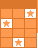Challenge Board: Score points by answering questions correctly.# Circumferance and Area of Circle Practice

Use you circle circumferance and area formulas to plug in the given information in each problem and to help you solve or find what each problem asks you to solve or find. In categories 5 and 6 you will plug in the information that is given in each problem, use the same circumferance and circle area formulas learned in class to find the radius or diameter. In categories 1-4 the radius or diameter was given and you needed to find the opposite or circumferance or area of the circle.MichelleHernandez

 This activity was created by a Quia Web subscriber. Learn more about QuiaCreate your own activities